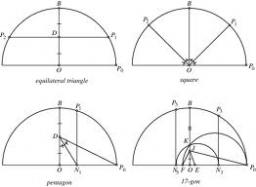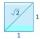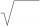# Numeric question

It is possible negative irrational number?

Result

#### Solution:

$-\sqrt{5}\left(\doteq -2.2360679775\right)\in I$We would be pleased if you find an error in the word problem, spelling mistakes, or inaccuracies and send it to us. Thank you!## Next similar math problems:

• LogsTrunk diameter is 52 cm. Is it possible to inscribe a square prism with side 36 cm?
• NumberIs number 5.146852 irrational?
• Expression 6Evaluate expression: -6-2(4-8)-9
• RootsCalculate the square root of these numbers:
• Transforming cuboidCuboid with dimensions 6 cm, 10, and 11 cm is converted into a cube with the same volume. What is its edge length?